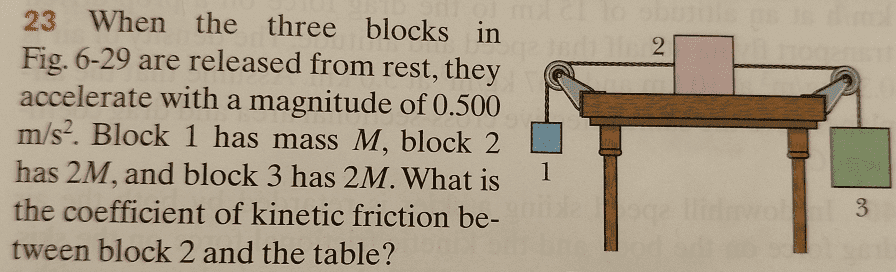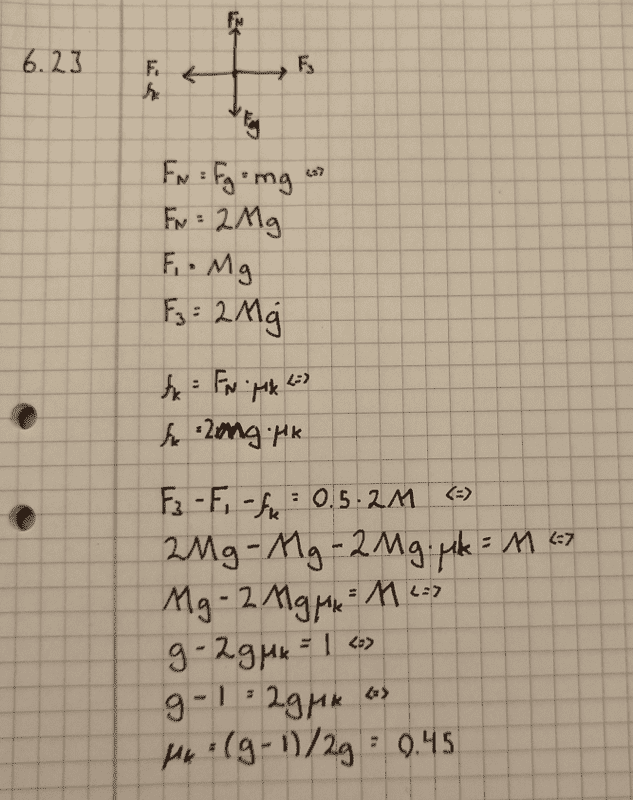# Find the kinetic friction coefficient

Homework Statement:
See image1.
Block 1: 1M kg
Block 2: 2M kg
Block 3: 2M kg
Resulting acceleration: .5 m/s2
Relevant Equations:
F = ma
fk = Fn * uImage1, the exercise.

My solution:Image2, my solution

The answer is .37 but I keep getting .45
What am I doing wrong?

collinsmark
Homework Helper
Gold Member
Homework Statement: See image1.
Block 1: 1M kg
Block 2: 2M kg
Block 3: 2M kg
Resulting acceleration: .5 m/s2
Homework Equations: F = ma
fk = Fn * u

View attachment 250394
Image1, the exercise.

My solution:
View attachment 250395
Image2, my solution

The answer is .37 but I keep getting .45
What am I doing wrong?
What's the total mass of the system?

5M but I don't see your point?
I use F=ma on the center block (block 2), what does the total mass have to do with anything?

haruspex
Homework Helper
Gold Member
2020 Award
what does the total mass have to do with anything?
All blocks will accelerate together, so their total mass is the total inertia.
Alternatively, assign unknowns to the tensions and draw a separate FBD for each block.

It produce the correct answer, but I still don't see the corelation.
I mean we only look at forces applied to Block 2, but for some reason we need to consider the mass of the two other blocks aswell?

I mean F (from F=ma) here is applied only to block 2, its not applied to block 1 or 3. If it were they'd all move sideways. How come m should include block1 and 3 then?

haruspex